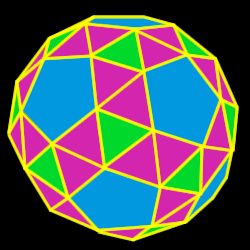# Snub Icosidodecahedron

Properties of the snub icosidodecahedron: Number of faces, edges and dihedral angle measure

The snub icosidodecahedron is created by either truncating (cutting off)
the dodecahedron or the icosahedron.92 total faces:
80 equilateral triangles and 12 regular pentagons

60 vertices:
4 triangles and 1 pentagon

150 edges

Dihedral angles
152 degrees, 16 minutes for the pent-tri angle and 164 degrees, 11 minutes for the tri-tri angle